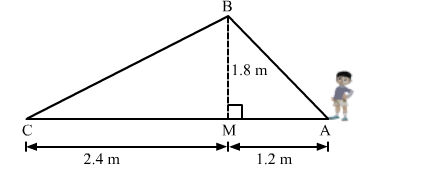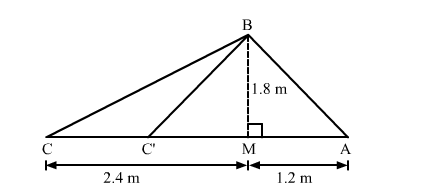# Naman is doing fly-fishing in a stream. The tip of his fishing rod is 1.8 m above the surface of the water and the fly at the end of the string rests on the water 3.6 mQuestion:

Naman is doing fly-fishing in a stream. The tip of his fishing rod is 1.8 m above the surface of the water and the fly at the end of the string rests on the water 3.6 m away from him and 2.4 m from the point directly under the tip of the road. Assuming that the string(from the top of his road to the fly) is taut, how much string does he have out (see the adjoining figure)? If he pulls in the string at the rate of 5 cm per second, what will be the horizontal distance of the fly from him after seconds.Solution:Naman pulls in the string at the rate of 5 cm per second.
Hence, after 12 seconds the length of the string he will pulled is given by
12 × 5 = 60 cm or 0.6 m
Now, In △BMC
By using Pythagoras theorem, we have
BC2 = CM2 + MB2
= (2.4)2 + (1.8)2
= 9
∴ BC = 3 m
Now, BC' = BC − 0.6
= 3 − 0.6
= 2.4 m
Now, In △BC'M
By using Pythagoras theorem, we have
C'M2 = BC'2 − MB2
= (2.4)2 − (1.8)2
= 2.52
∴ C'M = 1.6 m
The horizontal distance of the fly from him after 12 seconds is given by
C'A = C'M + MA
= 1.6 + 1.2
= 2.8 m